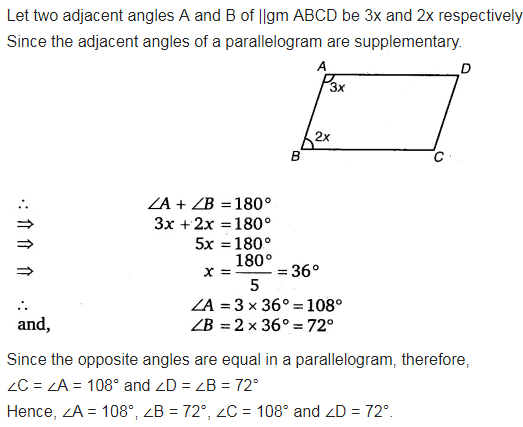# The measures of two adjacent angles of a parallelogram are in the ratio 3 :2

The measures of two adjacent angles of a parallelogram are in the ratio 3 :2. Find the measure of each of the angles of the parallelogram.